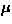Next: Detection of positrons Up: 2 The muon spin Previous: 2 The muon spin

## 2.1 The basics

TheSR technique is based on the two properties of muons:
(a)
muons are 100% spin-polarized, when produced from thedecay:(b)
muons decay into one positron and two neutrinos with a lifetime of=2.2s:The feature (a) results from the `parity violation' of the weak decays, namely, the fact that only left-handed neutrinos exist. As shown in Fig.6, the muon from thedecay has to be spin-polarized, in order to conserve the total spin zero of the pion.The feature (b) yields an asymmetric angular distribution of the decay-positrons relative to the muon spin direction. The angular distribution of decay positrons depends on their kinetic energy, which ranges from 0 to=53 MeV. The angular distribution is expressed as :(2)
where

andis the normalized positron energy,is the angle of positron emission measured from the muon spin direction, andis a small solid angle. After integrating the positron energy, the angular distribution behaves as shown in Fig.7.The basic idea of theSR technique follows the next three steps:

(1)
a muon is implanted in the sample with its spin polarized.
(2)
the muon spin changes its direction because of the magnetic environment around.
(3)
the muon decays into a positron and two neutrinos. The muon spin polarization at the time of the decay is reconstructed by measuring the positron directions for many incident muons.Next: Detection of positrons Up: 2 The muon spin Previous: 2 The muon spin